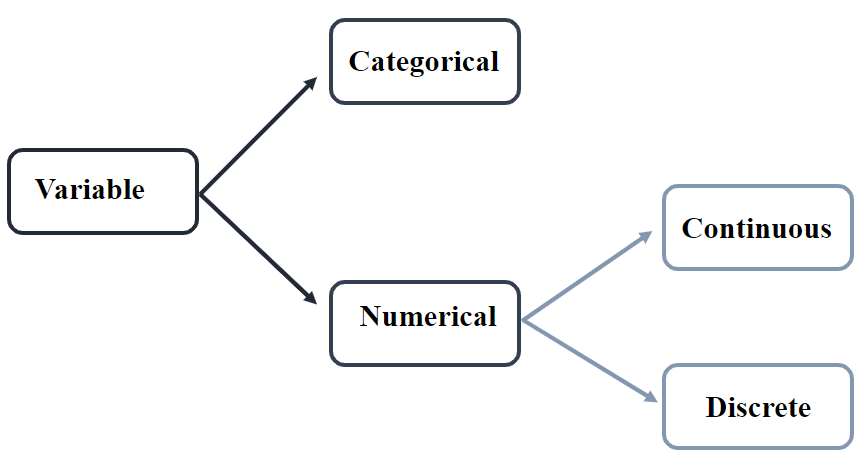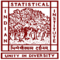Continuous Variable: How it is Different from Discrete Variable

# Continuous Variable: How it is Different from Discrete Variable

clickHere
Updated on Mar 6, 2023 15:44 IST

A numerical variable is said to be a continuous variable if it can take an infinite number of real values within a given interval or range. This article will discuss different types of numerical variables: Continuous and Discrete variables, their types, and difference between continuous and discrete variable.

Variables in mathematics are just a symbol that can represent different values in an expression, and they are mainly classified into Categorical and Numerical Variables.

• Categorical Variable is a variable that has two or more categories with no intrinsic ordering to the categories.
• It is also known as Qualitative Variables.
• Example: Sex, Age Group, Education Level
• Numerical Variable is a variable that can be either counted or measured.
• It is also known as quantitative Variables.
• Example: Height, Area, Distance, Time

Numerical Variables are further classified into discrete and Continuous Variables.## What is a Continuous Variable?

A numerical variable is said to be a continuous variable if it can take an infinite number of real values within a given interval or range.

• It can take positive, negative, interns, fractional, and decimal values.
• Continuous data can change over time.
• Continuous data can be best shown on a line graph.
• It is generally measured on a scale such as height, weight, and temperature.
• It is ideal for inferential statistics

Example:

1. Height of people living in the Asian Continent
2. Weight of the students of a school
3. The temperature of a city in a year
4. Speed of airplane
5. Area of a house

## Types of Continuous Variable:

Continuous Variables are broadly classified into:

• Interval Variable
• Ratio Variable

### Interval Variable

The interval variable is a measurement variable that is used to define values measured along a scale, such that each point are equidistant from the other.

• In simple terms, an interval variable is a variable where the difference between two points (or values) is meaningful.
• It has no zero value.

Example: Temperature (when measured in Celsius and Fahrenheit scale), Time, IQ Test Score, etc.

### Ratio Variable

• It is an extension of the Interval Variable, with the only difference being that the ratio variable already has zero.
• Unlike interval variables, here, you can perform multiplication and division operations.

Example: Temperature (when measured on Kelvin scale), Height, Weight, etc.

## What is a Discrete Variable

Variables that can only take a finite number of values are called a discrete variables.

• Variables whose values are obtained from counting are called Discrete Variables.
• All qualitative variables are discrete variables.

Example:

• Number of Wickets taken by a player
• Votes cast in an election
• Number of heads while tossing a coin 100 times

## Conclusion

Variables in mathematics or statistics are used to represent the values corresponding to its expression. Variables are broadly classified into Categorical and Numerical Variable. This article briefly discusses the numerical variable, its type with the help of examples. Later the article, also discusses the difference between Continuous and Discrete variables.

Top Trending Article

Interview Questions

clickHere

Vikram has a Postgraduate degree in Applied Mathematics, with a keen interest in Data Science and Machine Learning. He has experience of 2+ years in content creation in Mathematics, Statistics, Data Science, and Mac... Read Full Bio

## Trending Data Science Courses## Top Picks & New Arrivals